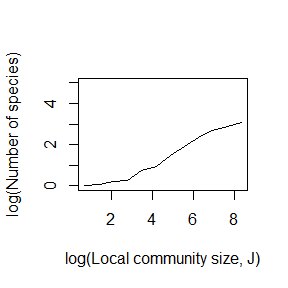## Online Box 8: R code to simulate the Theory of Island Biogeography: Figure 6.12 in the book

This code produces only one of the curves in Figure 6.12. The input csv file and the parameter m need to be altered in order to simulate the other scenarios. The input data used for the graph produced here was generated using nu <- 5e-04, J <- 10000 and num.years <- 10000 in Online Box 7.

## Import data on metacommunity relative abundance distribution
## produced by Online Box 7

## specify parameters, initial conditions, and output matrix
num.years <- 1000
J.min <- 2 # J on the smallest 'island'
num.J <- 12 # the number of islands (J values)
SR.meta = dim(metacom.data) # metacommunity species richness

m <- 0.01

SR.out <- matrix(nrow = (num.years+1), ncol = num.J); SR.out[1,] <- 1

## run simulations (one for each island)
for (j in 1:num.J) {
J <- J.min**j
COM <- vector(length = J); COM[] <- 1
time <- 2

## run individual simulation
for (i in 1:(J*num.years)) {

## immigration
if (runif(1) < m) {
COM[dead.indiv] <- sample(metacom.data[,1], size = 1, prob = metacom.data[,2])
} else {

## local reproduction
}

## record data
if (i %% J == 0) {
COM.hist <- hist(COM, c(1:SR.meta), plot=FALSE)
abund.vec <- COM.hist$counts[COM.hist$counts>0]
SR.out[time,j] <- sum(abund.vec>0)
time <- time + 1
}
} }

## graph the results (the average of the final 600 years of 'data')
plot(log(J.min**c(1:num.J)), log(colMeans(SR.out[400:1001,])), type="l",
xlab="log(Local community size, J)", ylab="log(Number of species)", ylim=c(0,5))### Description of the code.

1. This is the metacommunity relative abundance distribution produced by Online Box 7. You need to specify the directory and filename accordingly. Immigrants into a local patch are drawn from this distribution.
metacom.data <- read.csv("c:/temp/RAD1.csv", header = TRUE)
1. J.min and num.J determine the set of island (patch) sizes that will be simulated. The smallest patch has J.min individuals, and the largest has J.min**num.J (J.min raised to the power num.J), in this case 2**12 = 4096. The number of species in the metacommunity, SR.meta, helps with recording data later on.
J.min <- 2 # J on the smallest 'island'
num.J <- 12 # the number of islands (J values)
SR.meta = dim(metacom.data) # metacommunity species richness
1. This is where we set the community size for the patch being simulated. The community has J individuals, all of which are initially of species 1 (the most abundant in the metacommunity because metacom.data is rank ordered).
  J <- J.min**j
COM <- vector(length = J); COM[] <- 1
1. Immigration is simulated in a very similar way as speciation and dispersal in previous Boxes, except that when immigration happens, the probability of choosing a given species from the metacommunity is equal to its relative abundance. The species “identities” are in column 1 of metacom.data and their relative abundances are in column 2.
  if (runif(1) < m) {
COM[dead.indiv] <- sample(metacom.data[,1], size = 1, prob = metacom.data[,2])
} else {
1. We use the hist function as in Online Box 7 to calculate species richness at the end of a given year.
if (i %% J == 0) {
COM.hist <- hist(COM, c(1:SR.meta), plot=FALSE)
abund.vec <- COM.hist$counts[COM.hist$counts>0]
SR.out[time,j] <- sum(abund.vec>0)
time <- time + 1
}
} }
1. Because species richness ultimately fluctuates about an equilibrium value (set by J and m), we plot the average across the last 600 years of a given simulation (colMeans(SR.out[400:1001,])) as an estimate of the equilibrium value.
plot(log(J.min**c(1:num.J)), log(colMeans(SR.out[400:1001,])), type="l",
xlab="log(Local community size, J)", ylab="log(Number of species)", ylim=c(0,5))